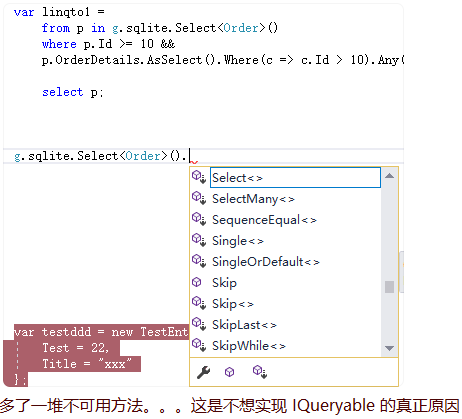# Linq to Sql

nicyeNovember 21, 2021About 2 minAbout 577 words

# # Linq to Sql

Originally not supporting IQueryable is mainly due to the consideration of usage habits. The intelligence of writing code will always prompt a bunch of methods you don't want to use. IQueryable itself provides a bunch of methods that cannot be implemented, as well as external intrusion extension methods, which seriously affect Coding experience. As shown below:For FreeSql v1.4.0+ version, please use the following items to install with commands (old version does not need to be installed):

dotnet add package FreeSql.Extensions.Linq

## # Special Note

• Please try not to use the following Linq methods in `ISelect` mode: `GroupJoin`, `Select`, `SelectMany`, `Join` and `DefaultIfEmpty`.

• If you must use the `.Select()` method in `ISelect`, be sure to call it before `.ToList()`.

## # IQueryable

FreeSql provides a powerful data query object: ISelect.

FreeSql.Extensions.Linq v1.4.0+ implements the common functions of IQueryable query objects for interactive use in various frameworks.

``````//Convert ISelect to IQueryable
IQueryable<Student> queryable = fsql.Select<Student>().AsQueryable();

//Linq Query
var t1 = queryable.Where(a => a.id == 1).FirstOrDefault();

//Restore IQueryable to ISelect
ISelect<Studeng> select = queryable.RestoreToSelect();
``````

Note: The implementation of IQueryable does not currently support `GroupBy`. You can consider using the `RestoreSelect` method to switch back to `ISelect` for query.

## # Where

``````var t1 = (
from a in fsql.Select<Student>()
where a.id == item.id
select a
).ToList();
``````

## # Specify Fields: Select

``````var t1 = (
from a in fsql.Select<Student>()
where a.id == item.id
select new { a.id }
).ToList();
``````

## # CaseWhen

``````var t1 = (
from a in fsql.Select<Student>()
where a.id == item.id
select new {
a.id,
a.name,
testsub = new {
time = a.age > 10 ? "大于" : "小于或等于"
}
}
).ToList();
``````

## # Join

``````var t1 = (
from a in fsql.Select<Student>()
join b in fsql.Select<School>() on a.id equals b.StudentId
select a
).ToList();

var t2 = (
from a in fsql.Select<Student>()
join b in fsql.Select<School>() on a.id equals b.StudentId
select new { a.id, bid = b.id }
).ToList();

var t3 = (
from a in fsql.Select<Student>()
join b in fsql.Select<School>() on a.id equals b.StudentId
where a.id == item.id
select new { a.id, bid = b.id }
).ToList();
``````

## # LeftJoin

``````var t1 = (
from a in fsql.Select<Student>()
join b in fsql.Select<School>() on a.id equals b.StudentId into temp
from tc in temp.DefaultIfEmpty()
select a
).ToList();

var t2 = (
from a in fsql.Select<Student>()
join b in fsql.Select<School>() on a.id equals b.StudentId into temp
from tc in temp.DefaultIfEmpty()
select new { a.id, bid = tc.id }
).ToList();

var t3 = (
from a in fsql.Select<Student>()
join b in fsql.Select<School>() on a.id equals b.StudentId into temp
from tc in temp.DefaultIfEmpty()
where a.id == item.id
select new { a.id, bid = tc.id }
).ToList();
``````

## # Multi-table Query: From

``````var t1 = (
from a in fsql.Select<Student>()
from b in fsql.Select<School>()
where a.id == b.StudentId
select a
).ToList();

var t2 = (
from a in fsql.Select<Student>()
from b in fsql.Select<School>()
where a.id == b.StudentId
select new { a.id, bid = b.id }
).ToList();

var t3 = (
from a in fsql.Select<Student>()
from b in fsql.Select<School>()
where a.id == b.StudentId
where a.id == item.id
select new { a.id, bid = b.id }
).ToList();
``````

## # Grouping: GroupBy

``````var t1 = (
from a in fsql.Select<Student>()
where a.id == item.id
group a by new {a.id, a.name } into g
select new {
g.Key.id, g.Key.name,
cou = g.Count(),
avg = g.Avg(g.Value.age),
sum = g.Sum(g.Value.age),
max = g.Max(g.Value.age),
min = g.Min(g.Value.age)
}
).ToList();
``````